# More Examples of Making Complex Heatmaps

Author: Zuguang Gu ( z.gu@dkfz.de )

Date: 2018-10-30

In the supplementaries of the ComplexHeatmap paper, there are four comprehensive examples which are applied on real-world high-throughput datasets. The examples can be found here.

Also my blog has some examples and tips for making better complex heatmaps.

Heatmaps are very popular to visualize gene expression matrix. Rows in the matrix correspond to genes and more information on these genes can be attached after the expression heatmap.

In following example, the big heatmap visualize relative expression for genes, then the next is the absolute expression. Also gene length and gene type (i.e. protein coding or lincRNA) are visualized.

``````library(ComplexHeatmap)
library(circlize)

expr = readRDS(paste0(system.file(package = "ComplexHeatmap"), "/extdata/gene_expression.rds"))
mat = as.matrix(expr[, grep("cell", colnames(expr))])
base_mean = rowMeans(mat)
mat_scaled = t(apply(mat, 1, scale))

type = gsub("s\\d+_", "", colnames(mat))
ha = HeatmapAnnotation(df = data.frame(type = type))

Heatmap(mat_scaled, name = "expression", km = 5, col = colorRamp2(c(-2, 0, 2), c("green", "white", "red")),
top_annotation = ha, top_annotation_height = unit(4, "mm"),
show_row_names = FALSE, show_column_names = FALSE) +
Heatmap(base_mean, name = "base_mean", show_row_names = FALSE, width = unit(5, "mm")) +
Heatmap(expr\$length, name = "length", col = colorRamp2(c(0, 1000000), c("white", "orange")),
heatmap_legend_param = list(at = c(0, 200000, 400000, 60000, 800000, 1000000),
labels = c("0kb", "200kb", "400kb", "600kb", "800kb", "1mb")),
width = unit(5, "mm")) +
Heatmap(expr\$type, name = "type", width = unit(5, "mm"))
``````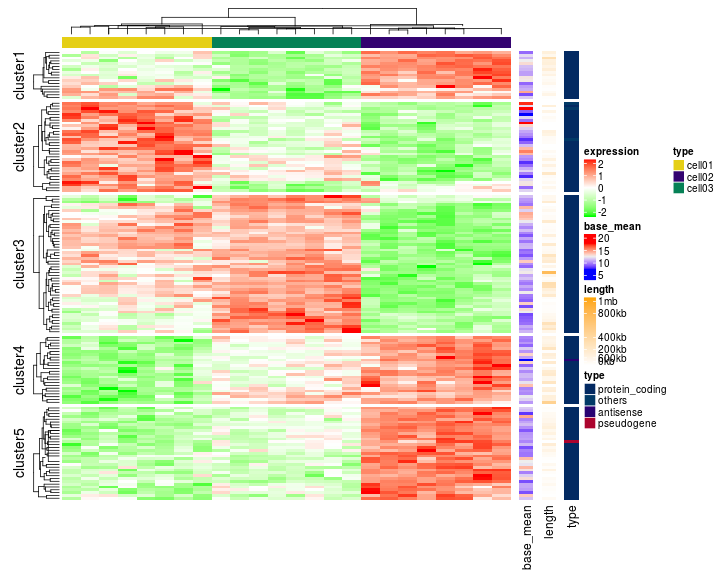### Visualize genomic regions and other correspondance

Following example visualizes correlation between methylation and expression, as well as other annotation information (data are randomly generated). In the heatmap, each row corresponds to a differentially methylated regions (DMRs). From left to right, heatmaps are:

1. methylation for each DMR (by rows) in samples.
2. direction of the methylation (one column heatmap), i.e. is methylation hyper in tumor or hypo?
3. expression for the genes that are associated with corresponding DMRs (e.g. closest gene).
4. significance for the correlation between methylation and expression (-log10(p-value)).
5. type of genes, i.e. is the gene a protein coding gene or a lincRNA?
6. annotation to gene models, i.e. is the DMR located in the intragenic region of the corresponding gene or the DMR is intergenic?
7. distance from the DMR to the TSS of the corresponding gene.
8. overlapping between DMRs and enhancers (Color shows how much the DMR is covered by the enhancers).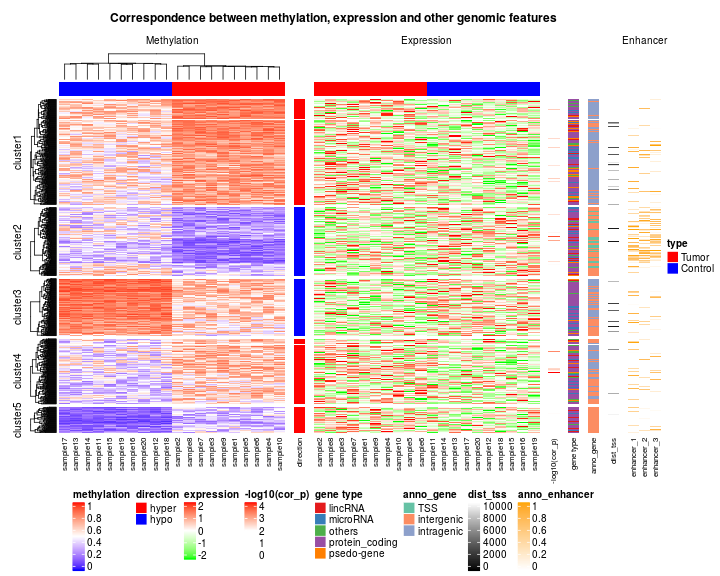## Combine pvclust and heatmap

pvclust package provides a robust way to test the stability of the clustering by random sampling from original data. Here you can organize the heatmap by the clustering returned from `pvclust()`.

``````library(ComplexHeatmap)

library(MASS)
library(pvclust)
data(Boston)
boston.pv <- pvclust(Boston, nboot=100)
``````
``````## Bootstrap (r = 0.5)... Done.
## Bootstrap (r = 0.6)... Done.
## Bootstrap (r = 0.7)... Done.
## Bootstrap (r = 0.8)... Done.
## Bootstrap (r = 0.9)... Done.
## Bootstrap (r = 1.0)... Done.
## Bootstrap (r = 1.1)... Done.
## Bootstrap (r = 1.2)... Done.
## Bootstrap (r = 1.3)... Done.
## Bootstrap (r = 1.4)... Done.
``````
``````plot(boston.pv)
``````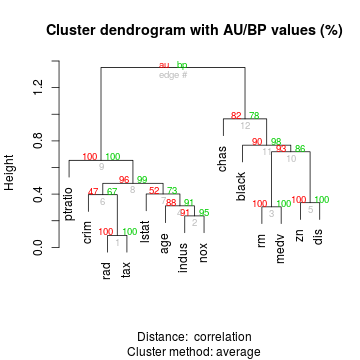Since by default `pvclust` clusters columns by 'correlation' method, we scale columns for `Boston` data set to see the relative trend.

``````Boston_scaled = apply(Boston, 2, scale)
Heatmap(Boston_scaled, cluster_columns = boston.pv\$hclust, heatmap_legend_param = list(title = "Boston"))
``````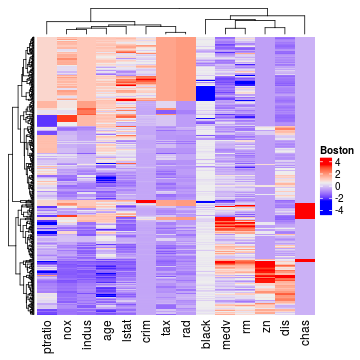## Make a same plot as heatmap()

``````set.seed(123)
mat = matrix(rnorm(100), 10)
heatmap(mat, col = topo.colors(50))
``````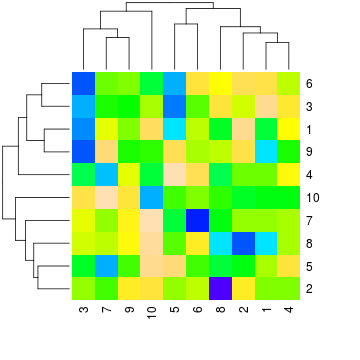Compare to the native `heatmap()`, `Heatmap()` can give more accurate interpolation for colors for continous values.

``````Heatmap(mat, col = topo.colors(50), color_space = "sRGB",
row_dend_width = unit(2, "cm"),
column_dend_height = unit(2, "cm"), row_dend_reorder = TRUE,
column_dend_reorder = TRUE)
``````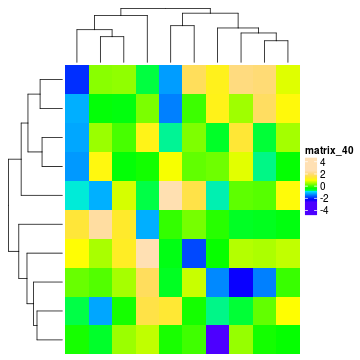## The measles vaccine heatmap

Following code reproduces the heatmap introduced here and here.

``````mat = readRDS(paste0(system.file("extdata", package = "ComplexHeatmap"), "/measles.rds"))
ha1 = HeatmapAnnotation(dist1 = anno_barplot(colSums(mat), bar_width = 1, gp = gpar(col = NA, fill = "#FFE200"),
border = FALSE, axis = TRUE))
ha2 = rowAnnotation(dist2 = anno_barplot(rowSums(mat), bar_width = 1, gp = gpar(col = NA, fill = "#FFE200"),
border = FALSE, which = "row", axis = TRUE), width = unit(1, "cm"))
ha_column = HeatmapAnnotation(cn = function(index) {
year = as.numeric(colnames(mat))
which_decade = which(year %% 10 == 0)
grid.text(year[which_decade], which_decade/length(year), 1, just = c("center", "top"))
})
Heatmap(mat, name = "cases", col = colorRamp2(c(0, 800, 1000, 127000), c("white", "cornflowerblue", "yellow", "red")),
cluster_columns = FALSE, show_row_dend = FALSE, rect_gp = gpar(col= "white"), show_column_names = FALSE,
row_names_side = "left", row_names_gp = gpar(fontsize = 10),
column_title = 'Measles cases in US states 1930-2001\nVaccine introduced 1961',
top_annotation = ha1, top_annotation_height = unit(1, "cm"),
bottom_annotation = ha_column, bottom_annotation_height = grobHeight(textGrob("1900"))) + ha2

decorate_heatmap_body("cases", {
i = which(colnames(mat) == "1961")
x = i/ncol(mat)
grid.lines(c(x, x), c(0, 1), gp = gpar(lwd = 2))
grid.text("Vaccine introduced", x, unit(1, "npc") + unit(5, "mm"))
})
``````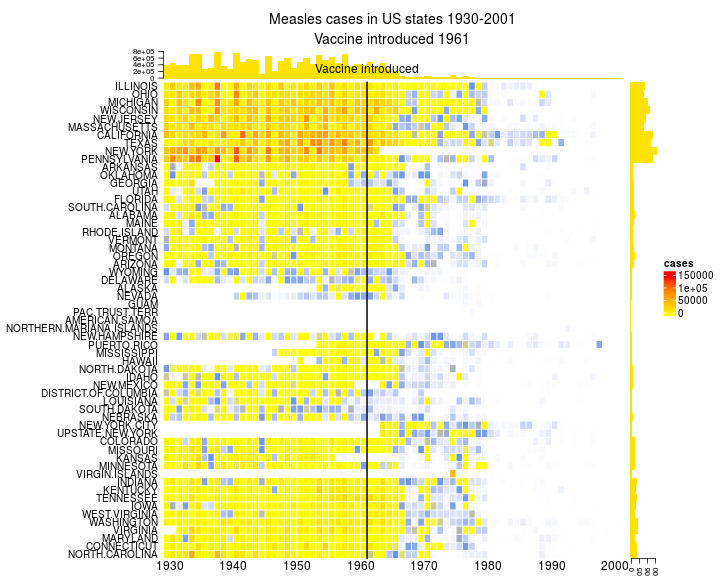## What if my annotation name is too long?

There is no space allocated for annotation name, but when the annotation name is too long, you can add paddings of the whole plot to give empty spaces for the annotation names.

``````ha = HeatmapAnnotation(df = data.frame(a_long_long_long_annotation_name = runif(10)),
show_legend = FALSE)
ht = Heatmap(matrix(rnorm(100), 10), name = "foo", top_annotation = ha)
# because the default width for row cluster is 1cm
padding = unit.c(unit(2, "mm"), grobWidth(textGrob("a_long_long_long_annotation_name")) - unit(1, "cm"),
unit(c(2, 2), "mm"))
decorate_annotation("a_long_long_long_annotation_name", {
grid.text("a_long_long_long_annotation_name", 0, 0.5, just = "right")
})
``````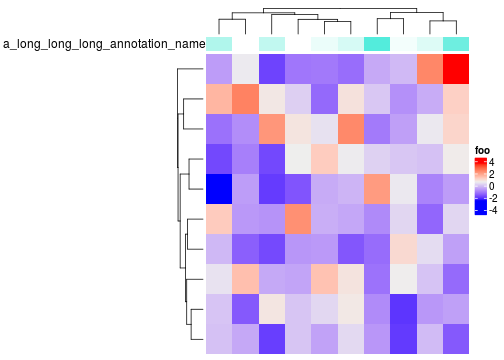## Session info

``````sessionInfo()
``````
``````## R version 3.5.1 Patched (2018-07-12 r74967)
## Platform: x86_64-pc-linux-gnu (64-bit)
## Running under: Ubuntu 16.04.5 LTS
##
## Matrix products: default
## BLAS: /home/biocbuild/bbs-3.8-bioc/R/lib/libRblas.so
## LAPACK: /home/biocbuild/bbs-3.8-bioc/R/lib/libRlapack.so
##
## locale:
##   LC_CTYPE=en_US.UTF-8       LC_NUMERIC=C               LC_TIME=en_US.UTF-8
##   LC_COLLATE=C               LC_MONETARY=en_US.UTF-8    LC_MESSAGES=en_US.UTF-8
##   LC_PAPER=en_US.UTF-8       LC_NAME=C                  LC_ADDRESS=C
##  LC_TELEPHONE=C             LC_MEASUREMENT=en_US.UTF-8 LC_IDENTIFICATION=C
##
## attached base packages:
##   stats4    parallel  grid      stats     graphics  grDevices utils     datasets  methods
##  base
##
## other attached packages:
##   pvclust_2.0-0         MASS_7.3-51           RColorBrewer_1.1-2    GetoptLong_0.1.7
##   dendextend_1.9.0      dendsort_0.3.3        cluster_2.0.7-1       IRanges_2.16.0
##   S4Vectors_0.20.0      BiocGenerics_0.28.0   HilbertCurve_1.12.0   circlize_0.4.4
##  ComplexHeatmap_1.20.0 knitr_1.20            markdown_0.8
##
## loaded via a namespace (and not attached):
##   mclust_5.4.1           Rcpp_0.12.19           mvtnorm_1.0-8          lattice_0.20-35
##   png_0.1-7              class_7.3-14           assertthat_0.2.0       mime_0.6
##   R6_2.3.0               GenomeInfoDb_1.18.0    plyr_1.8.4             evaluate_0.12
##  ggplot2_3.1.0          highr_0.7              pillar_1.3.0           GlobalOptions_0.1.0
##  zlibbioc_1.28.0        rlang_0.3.0.1          lazyeval_0.2.1         diptest_0.75-7
##  kernlab_0.9-27         whisker_0.3-2          stringr_1.3.1          RCurl_1.95-4.11
##  munsell_0.5.0          compiler_3.5.1         pkgconfig_2.0.2        shape_1.4.4
##  nnet_7.3-12            tidyselect_0.2.5       gridExtra_2.3          tibble_1.4.2
##  GenomeInfoDbData_1.2.0 viridisLite_0.3.0      crayon_1.3.4           dplyr_0.7.7
##  bitops_1.0-6           gtable_0.2.0           magrittr_1.5           scales_1.0.0
##  stringi_1.2.4          XVector_0.22.0         viridis_0.5.1          flexmix_2.3-14
##  bindrcpp_0.2.2         robustbase_0.93-3      fastcluster_1.1.25     HilbertVis_1.40.0
##  rjson_0.2.20           tools_3.5.1            fpc_2.1-11.1           glue_1.3.0
##  trimcluster_0.1-2.1    DEoptimR_1.0-8         purrr_0.2.5            colorspace_1.3-2
##  GenomicRanges_1.34.0   prabclus_2.2-6         bindr_0.1.1            modeltools_0.2-22
``````